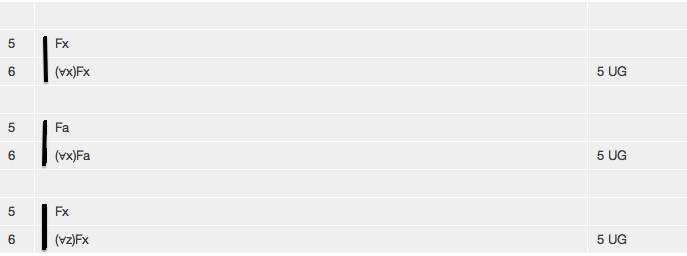# Tutorial 19: Universal Generalization

Logical System

2013

### The Tutorial

There also is a rule for adding a Universal Quantifier. This permits the step illustrated by the following proof fragments.The rule is quite simple

#### The Rule of Universal Generalization UG

If a derivation contains a line of the form

n. <formula> <any justification>

then a line of the form

(∀<variable>)<formula> 'n UG'

may be added to the derivation, provided that <variable> is not free in the <assumptions> of the formula that is generalized (the formula of line n).

You will notice that there is a restriction on the rule-- what this is, and why it is there, will be considered in Tutorial 23.

## Exercise to accompany Predicate Tutorial 9

### Exercise 1(of 1)

Derive the following

(a) Fa ∴ (∀x)Fa
(b) (∀x) (Fx∧Gx) ∴ (∀x)Fx
(c) (∀x) (Fx∧Gx) ∴ (∀y)Fy
(d) ∴ (∀x)Fx≡(∀y)Fy
(e) (∀x) (Fx∧Gx) ∴ (∀y)Fy∧(∀z)Gz
(f) ∴ (∀x) (Fx∧Gx) ≡ (∀y)Fy∧(∀z)Gz
(g) ∴ (∀x) (Fx∧Gx) ≡ (∀x)Fx∧(∀x)Gx